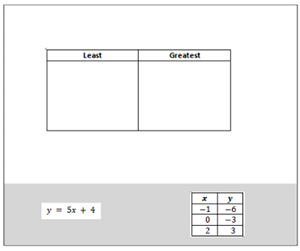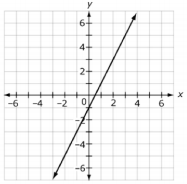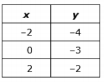# MAFS.8.F.1.2

Compare properties of two functions each represented in a different way (algebraically, graphically, numerically in tables, or by verbal descriptions). For example, given a linear function represented by a table of values and a linear function represented by an algebraic expression, determine which function has the greater rate of change.

### Clarifications

Examples of Opportunities for In-Depth Focus

Work toward meeting this standard repositions previous work with tables and graphs in the new context of input/output rules.
General Information
Subject Area: Mathematics
Domain-Subdomain: Functions
Cluster: Level 2: Basic Application of Skills & Concepts
Cluster: Define, evaluate, and compare functions. (Major Cluster) -

Clusters should not be sorted from Major to Supporting and then taught in that order. To do so would strip the coherence of the mathematical ideas and miss the opportunity to enhance the major work of the grade with the supporting clusters.

Date of Last Rating: 02/14
Status: State Board Approved
Assessed: Yes
Test Item Specifications

• Assessment Limits :
Function notation may not be used. Functions must be linear.
• Calculator :

Yes

• Context :

Allowable

Sample Test Items (2)
• Test Item #: Sample Item 1
• Question:

Drag each function to the box to show the least rate and the greatest rate.• Difficulty: N/A
• Type: GRID: Graphic Response Item Display

• Test Item #: Sample Item 2
• Question:

Two linear functions are shown.

Function 1:Function 2:Create an equation for a third function that has a greater rate of change than one function but a smaller rate of change than the other function.

• Difficulty: N/A
• Type: EE: Equation Editor

## Related Courses

This benchmark is part of these courses.
1205050: M/J Accelerated Mathematics Grade 7 (Specifically in versions: 2014 - 2015, 2015 - 2020, 2020 - 2022 (current), 2022 and beyond)
1205070: M/J Grade 8 Pre-Algebra (Specifically in versions: 2014 - 2015, 2015 - 2022 (current), 2022 and beyond)
1204000: M/J Foundational Skills in Mathematics 6-8 (Specifically in versions: 2014 - 2015, 2015 - 2022 (current), 2022 and beyond)
7812030: Access M/J Grade 8 Pre-Algebra (Specifically in versions: 2014 - 2015, 2015 - 2018, 2018 - 2019, 2019 and beyond (current))

## Related Access Points

Alternate version of this benchmark for students with significant cognitive disabilities.
MAFS.8.F.1.AP.2a: Compare the rise/run (m) of two simple linear functions.

## Related Resources

Vetted resources educators can use to teach the concepts and skills in this benchmark.

## Assessments

Sample 4 - Eighth Grade Math State Interim Assessment:

This is a State Interim Assessment for eighth grade.

Type: Assessment

Sample 3 - Eighth Grade Math State Interim Assessment:

This is a State Interim Assessment for eighth grade.

Type: Assessment

Sample 2 - Eighth Grade Math State Interim Assessment:

This is a State Interim Assessment for eighth grade.

Type: Assessment

Sample 1 - Eighth Grade Math State Interim Assessment:

This is a State Interim Assessment for eighth grade.

Type: Assessment

## Formative Assessments

Interpreting Distance-Time Graphs:

This lesson unit is intended to help you assess how well students are able to interpret distance-time graphs and, in particular, to help you identify students who:

• Interpret distance-time graphs as if they are pictures of situations rather than abstract representations of them.
• Have difficulty relating speeds to slopes of these graphs.

Type: Formative Assessment

Innovative Functions:

Students are asked to determine the rates of change of two functions presented in different forms (an expression and a table) and determine which is the greater rate of change within a real-world context.

Type: Formative Assessment

Competing Functions:

Students are asked to determine and interpret the initial values of two functions represented in different ways (equation and graph), and compare them.

Type: Formative Assessment

Students are asked to determine the rate of change of two functions presented in different forms (table and graph) and determine which is the greater rate of change within a real-world context.

Type: Formative Assessment

This House Is Mine!:

Students are asked to determine a specific value of two functions given in different forms (a graph and a verbal description) within a real-world context, and compare them.

Type: Formative Assessment

## Lesson Plans

Select a Healthcare Plan:

Students are asked to determine a procedure for ranking healthcare plans based on their assumptions and the cost of each plan given as a function. Then, they are asked to revise their ranking based on a new set of data.

Type: Lesson Plan

Lines and Linear Equations:

This lesson unit is intended to help you assess how well students are able to interpret speed as the slope of a linear graph and translate between the equation of a line and its graphical representation.

Type: Lesson Plan

The Linear Function Connection:

The student will compare two linear functions that have been represented in different ways (equation, table, graph, verbal description). They will be able to find and compare the rate of change of the function from any of the representations.

Type: Lesson Plan

How much is My Cell Phone Plan?:

In this lesson, shopping for cell phone plans provides a motivating context where students will compare and interpret the properties of two functions represented in different ways. The lesson starts with students examining and discussing an input/output table to identify rate of change and y-intercept. Students will then be hooked by the talk of cell phones. Students will be assigned a group. Each group will solve two different problems involving cell phone plans. They have to determine the rate of change, y-intercept, and the total cost after two years. Students will be assigned homework and have a summative assessment the next day.

Type: Lesson Plan

## Original Student Tutorial

Functions, Sweet Functions:

See how sweet it can be to determine the slope of linear functions and compare them in this interactive tutorial. Determine and compare the slopes or the rates of change by using verbal descriptions, tables of values, equations and graphical forms.

Type: Original Student Tutorial

Battery Charging:

This task has students engaging in a simple modeling exercise, taking verbal and numerical descriptions of battery life as a function of time and writing down linear models for these quantities. To draw conclusions about the quantities, students have to find a common way of describing them.

## Student Center Activity

Students can practice answering mathematics questions on a variety of topics. With an account, students can save their work and send it to their teacher when complete.

Type: Student Center Activity

## Unit/Lesson Sequence

Linear Functions and Slope:

This session on linear function and slope contains five parts, multiple problems and videos, and interactive activities geared to help students recognize and understand linear relationships, explore slope and dependent and independent variables in graphs of linear relationships, and develop an understanding of rates and how they are related to slopes and equations. Throughout the session, students use spreadsheets to complete the work, and are encouraged to think about the ways technology can aid in teaching and understanding. The solutions for all problems are given, and many allow students to have a hint or tip as they solve. There is even a homework assignment with four problems for students after they have finished all five parts of the session.

Type: Unit/Lesson Sequence

## STEM Lessons - Model Eliciting Activity

Select a Healthcare Plan:

Students are asked to determine a procedure for ranking healthcare plans based on their assumptions and the cost of each plan given as a function. Then, they are asked to revise their ranking based on a new set of data.

## MFAS Formative Assessments

Competing Functions:

Students are asked to determine and interpret the initial values of two functions represented in different ways (equation and graph), and compare them.

Innovative Functions:

Students are asked to determine the rates of change of two functions presented in different forms (an expression and a table) and determine which is the greater rate of change within a real-world context.

Students are asked to determine the rate of change of two functions presented in different forms (table and graph) and determine which is the greater rate of change within a real-world context.

This House Is Mine!:

Students are asked to determine a specific value of two functions given in different forms (a graph and a verbal description) within a real-world context, and compare them.

## Original Student Tutorials Mathematics - Grades 6-8

Functions, Sweet Functions:

See how sweet it can be to determine the slope of linear functions and compare them in this interactive tutorial. Determine and compare the slopes or the rates of change by using verbal descriptions, tables of values, equations and graphical forms.

## Student Resources

Vetted resources students can use to learn the concepts and skills in this benchmark.

## Original Student Tutorial

Functions, Sweet Functions:

See how sweet it can be to determine the slope of linear functions and compare them in this interactive tutorial. Determine and compare the slopes or the rates of change by using verbal descriptions, tables of values, equations and graphical forms.

Type: Original Student Tutorial

## Student Center Activity

Students can practice answering mathematics questions on a variety of topics. With an account, students can save their work and send it to their teacher when complete.

Type: Student Center Activity

## Parent Resources

Vetted resources caregivers can use to help students learn the concepts and skills in this benchmark.